Author: Oscar Cronquist Article last updated on May 05, 2022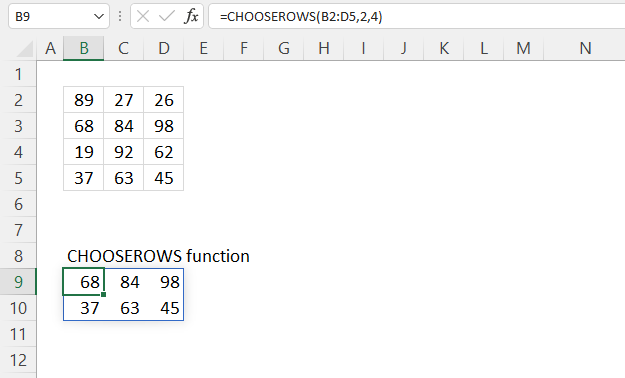The CHOOSEROWS function returns given rows from a cell range or array.

Dynamic array formula in cell B9:

=CHOOSEROWS(B2:D5, 2, 4)

The CHOOSEROWS function is available to Excel 365 users and is in the "Array manipulation" category.

## 1. CHOOSEROWS Function Syntax

CHOOSEROWS(array, row_num1, [row_num2], …)

## 2. CHOOSEROWS Function Arguments

 array Required. The source cell range or array. row_num1 Required. A number representing the row in a given cell range. [row_num2] Optional. Extra row numbers to be extracted.

## 3. CHOOSEROWS Function example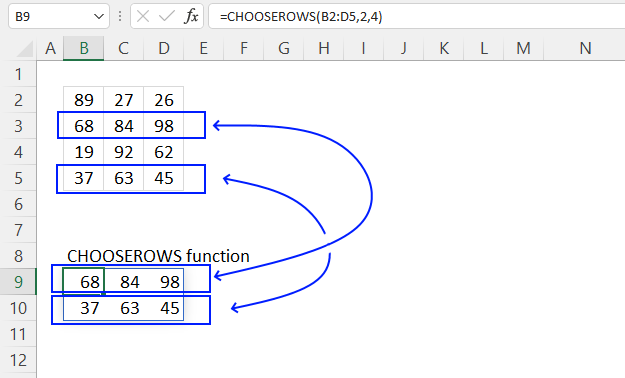The picture above shows how the CHOOSEROWS function extracts the second and fourth row from cell range B2:D5.

Dynamic array formula in cell B9:

=CHOOSEROWS(B2:D5, 2, 4)
Note! If any of the values in the source range change, the values in the CHOOSEROWS function output change as well.

### 3.1 Explaining formula

#### Step 1 - CHOOSEROWS function

CHOOSEROWS(array, row_num1, [row_num2], …)

array - B2:D5
row_num1 - 2
[row_num2] - 4

#### Step 3 - Evaluate function

CHOOSEROWS(B2:D5, 2, 4)

becomes

CHOOSEROWS({89, 27, 26;
68, 84, 98;
19, 92, 62;
37, 63, 45}, 2)

and returns

{68, 84, 98;
37, 63, 45}

## 4. CHOOSEROWS Function example - array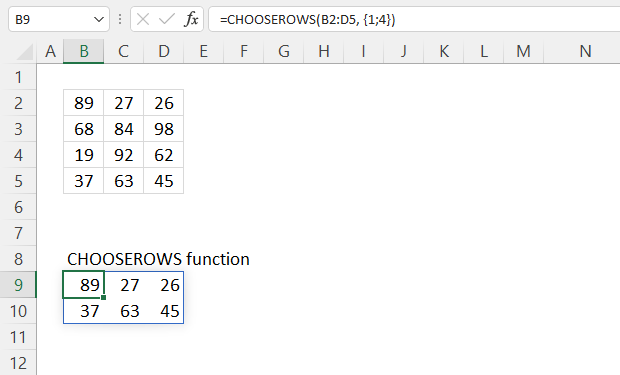The CHOOSEROWS function lets you use an array in the arguments, the image above shows an array in the first argument.

Dynamic array formula in cell B9:

=CHOOSEROWS(B2:D5, {1; 4})

### Explaining formula

#### Step 1 - Populate the array

The curly brackets let you build an array that you can use in most but not all Excel functions. The ; semicolon is a delimiting character that separates values row by row.

The CHOOSE function requires numbers separated with semicolons or whatever row delimiting character you use.

{1; 4}

#### Step 2 - CHOOSEROWS function

CHOOSEROWS(array, row_num1, [row_num2], …)

CHOOSEROWS(B2:D5, {1; 4})

becomes

CHOOSEROWS({89, 27, 26;
68, 84, 98;
19, 92, 62;
37, 63, 45}, {1; 4})

and returns

{89, 27, 26;
37, 63, 45}

## 5. CHOOSEROWS Function example - split text to array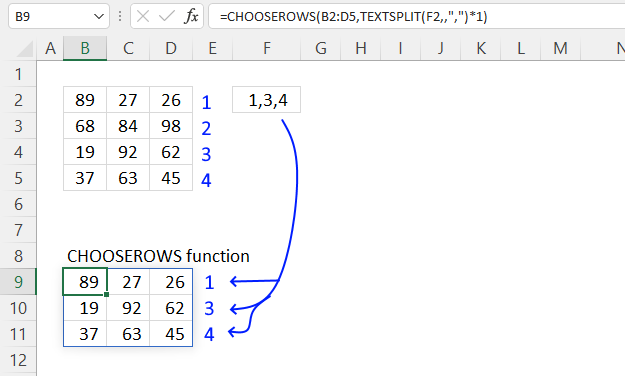The section shows how to return specific rows based on a string containing numbers. The TEXTSPLIT function separates

Dynamic array formula in cell B9:

=CHOOSEROWS(B2:D5,TEXTSPLIT(F2,,",")*1)

### Explaining formula

#### Step 1 - Split text

The TEXTSPLIT function lets you split a string into an array across columns and rows based on delimiting characters.

TEXTSPLIT(Input_Textcol_delimiter, [row_delimiter], [Ignore_Empty])

TEXTSPLIT(F2,,",")

becomes

TEXTSPLIT("1,3,4",,",")

and returns {"1"; "3"; "4"}. Note the double quotes, Excel handles these values as text values. The next step converts text values to numbers.

#### Step 2 - Convert text to numbers

The asterisk lets you multiply numbers in an Excel formula, I am using it here to convert text to numbers.

TEXTSPLIT(F2,,",")*1

becomes

{"1"; "3"; "4"}*1

and returns {1; 3; 4}.

#### Step 3 - CHOOSEROWS function

CHOOSEROWS(B2:D5,TEXTSPLIT(F2,,",")*1)

becomes

CHOOSEROWS(B2:D5, {1; 3; 4})

becomes

CHOOSEROWS({89, 27, 26;
68, 84, 98;
19, 92, 62;
37, 63, 45}, {1; 3; 4})

and returns

{89,27,26;
19,92,62;
37,63,45}

## 6. CHOOSEROWS Function example - negative values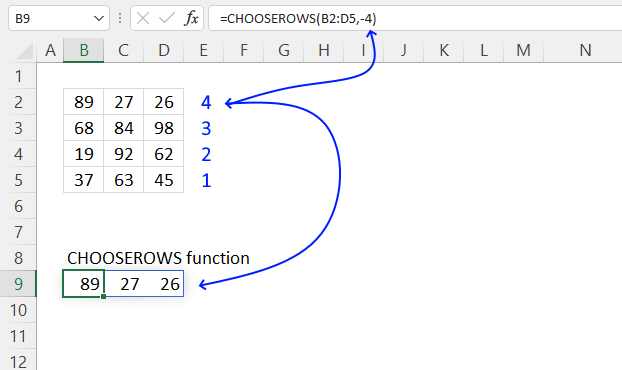The CHOOSEROWS function lets you use negative numbers, this will make the function count from the bottom or from the end.

Formula in cell B9:

=CHOOSEROWS(B2:D5, -4)

CHOOSEROWS(array, row_num1, [row_num2], …)

## 7. CHOOSEROWS function error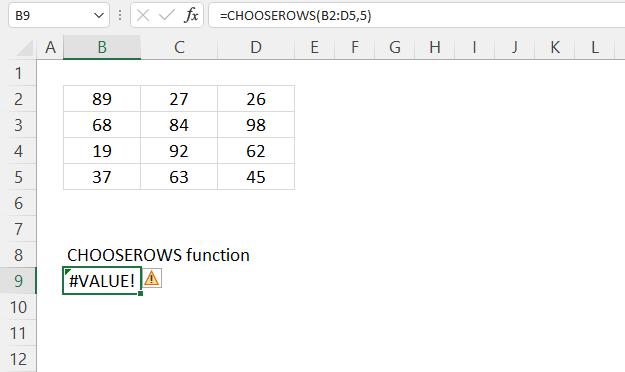The CHOOSEROWS function returns a #VALUE! error when no values can be displayed.

## 8. CHOOSEROWS function - values in random order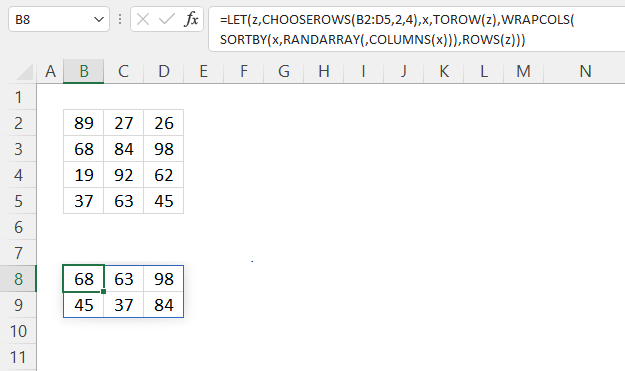The image above shows a formula that returns values in random order from the two first rows in a 2D cell range.

Dynamic array formula in cell B8:

=LET(z, CHOOSEROWS(B2:D5, 2), x, TOROW(z), WRAPCOLS(SORTBY(x, RANDARRAY(, COLUMNS(x))), ROWS(z)))

### 8.1 Explaining formula in cell B8

#### Step 1 - Remove two first rows

CHOOSEROWS(B2:D5, 2, 4)

becomes

CHOOSEROWS({89, 27, 26;
68, 84, 98;
19, 92, 62;
37, 63, 45}, 2, 4)

and returns

{68,84,98;
37,63,45}.

#### Step 2 - Rearrange values to a single row

The TOROW function rearranges values from a 2D cell range or array to a single row.

TOROW(array, [ignore], [scan_by_col])

TOROW(CHOOSEROWS(B2:D5,2,4))

becomes

TOROW({68,84,98;
37,63,45})

and returns

{68, 84, 98; 37, 63, 45}.

#### Step 3 - Count cells

The COLUMNS function returns the number of columns in a given cell range or array.

COLUMNS(array)

COLUMNS(TOROW(CHOOSEROWS(B2:D5,2, 4)))

becomes

COLUMNS({68, 84, 98, 37, 63, 45})

and returns 6.

#### Step 4 - Count rows

The ROWS function returns the number of rows in a given cell range or array.

ROWS (array)

ROWS(CHOOSEROWS(B2:D5,2, 4))

becomes

ROWS({68, 84, 98; 37, 63, 45})

and returns 2.

#### Step 5 - Create random decimal numbers

The RANDARRAY function returns a table of random numbers across rows and columns.

RANDARRAY([rows], [columns], [min], [max], [whole_number])

RANDARRAY(,COLUMNS(TOROW(CHOOSEROWS(B2:D5,2, 4))))

becomes

RANDARRAY(,6)

and returns

{0.215398134613085, 0.390607168196479, ... ,0.83231474462401}.

#### Step 6 - Rearrange values in random order

The SORTBY function allows you to sort values from a cell range or array based on a corresponding cell range or array.

SORTBY(array, by_array1, [sort_order1], [by_array2, sort_order2],…)

SORTBY(TOROW(CHOOSEROWS(B2:D5,2, 4)),RANDARRAY(,COLUMNS(CHOOSEROWS(B2:D5,2, 4))))

becomes

SORTBY({68, 84, 98, 37, 63, 45}, {0.215398134613085, 0.390607168196479, ... ,0.83231474462401})

and returns

{63, 37, 45, 98, 84, 68}

#### Step 7 - Rearrange values to the original array size

The WRAPCOLS function rearranges values from a single row to a 2D cell range based on a given number of values per column.

WRAPCOLS(SORTBY(TOROW(CHOOSEROWS(B2:D5,2, 4)),RANDARRAY(,COLUMNS(TOROW(CHOOSEROWS(B2:D5,2, 4))))),ROWS(CHOOSEROWS(B2:D5,2, 4)))

becomes

WRAPCOLS({63, 37, 45, 98, 84, 68}, ROWS(CHOOSEROWS(B2:D5,2, 4)))

becomes

WRAPCOLS({63, 37, 45, 98, 84, 68}, 2)

and returns

{63, 37, 45; 98, 84, 68}.

#### Step 8 - Shorten formula

The LET function lets you name intermediate calculation results which can shorten formulas considerably and improve performance.

LET(name1name_value1calculation_or_name2, [name_value2calculation_or_name3...])

WRAPCOLS(SORTBY(TOROW(CHOOSEROWS(B2:D5, 2, 4)), RANDARRAY(, COLUMNS(TOROW(CHOOSEROWS(B2:D5, 2, 4))))), TOROW(CHOOSEROWS(B2:D5, 2, 4)))

becomes

LET(z, CHOOSEROWS(B2:D5, 2, 4), x, TOROW(z), WRAPCOLS(SORTBY(x, RANDARRAY(, COLUMNS(x))), ROWS(z)))

## 9. CHOOSEROWS Function - multiple source ranges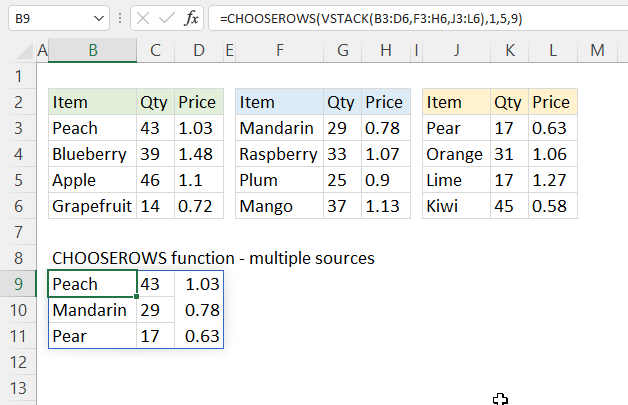The picture above shows a formula that merges three non-contiguous cell ranges and shows rows 1, 5, and 9.

Dynamic array formula in cell B9:

=CHOOSEROWS(VSTACK(B3:D6, F3:H6, J3:L6), 1, 5, 9)

### 9.1 Explaining formula

#### Step 1 - Stack values horizontally

The VSTACK function lets you combine cell ranges or arrays, it joins data to the first blank cell at the bottom of a cell range or array (vertical stacking)

VSTACK(array1, [array2],...)

VSTACK(B3:D6, F3:H6, J3:L6)

becomes

VSTACK({"Peach", 43, 1.03;"Blueberry", 39, 1.48;"Apple", 46, 1.1;"Grapefruit", 14, 0.72}, {"Mandarin", 29, 0.78;"Raspberry", 33, 1.07;"Plum", 25, 0.9;"Mango", 37, 1.13}, {"Pear", 17, 0.63;"Orange", 31, 1.06;"Lime", 17, 1.27;"Kiwi", 45, 0.58})

and returns

{"Peach", 43, 1.03;
"Blueberry", 39, 1.48;
"Apple", 46, 1.1;
"Grapefruit", 14, 0.72;
"Mandarin", 29, 0.78;
"Raspberry", 33, 1.07;
"Plum", 25, 0.9;
"Mango", 37, 1.13;
"Pear", 17, 0.63;
"Orange", 31, 1.06;
"Lime", 17, 1.27;
"Kiwi", 45, 0.58}

#### Step 2 - Filter given rows

CHOOSEROWS(VSTACK(B3:D6, F3:H6, J3:L6),1, 5, 9)

becomes

CHOOSEROWS({"Peach", 43, 1.03;
"Blueberry", 39, 1.48;
"Apple", 46, 1.1;
"Grapefruit", 14, 0.72;
"Mandarin", 29, 0.78;
"Raspberry", 33, 1.07;
"Plum", 25, 0.9;
"Mango", 37, 1.13;
"Pear", 17, 0.63;
"Orange", 31, 1.06;
"Lime", 17, 1.27;
"Kiwi", 45, 0.58}, 1, 5, 9)

and returns

{"Peach", 43, 1.03;
"Mandarin", 29, 0.78;
"Pear", 17, 0.63}

## 10. CHOOSEROWS Function - get every other row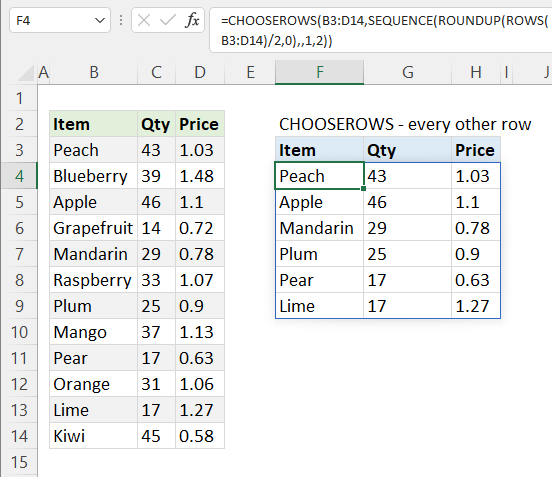The formula in cell F4 extracts every other row in cell range B3:D14, the dynamic array formula calculates how many rows are needed automatically based on the cell reference.

Formula in cell F4:

=CHOOSEROWS(B3:D14, SEQUENCE(ROUNDUP(ROWS(B3:D14)/2,0), , 1, 2))

### 10.1 Explaining formula

#### Step 1 - Count rows

The ROWS function returns a number corresponding to the number of rows in a given cell reference.

ROWS(array)

ROWS(B3:D14)

returns 12. There are twelve rows in cell reference B3:D14.

#### Step 2 - Round number up

The ROUNDUP function rounds a number up.

ROUNDUP(numbernum_digits)

ROUNDUP(ROWS(B3:D14)/2,0)

becomes

ROUNDUP(12/2,0)

becomes

ROUNDUP(6,0)

and returns 6.

#### Step 3 - Create a sequence of numbers from 1 to n step 2

The SEQUENCE function creates a list of sequential numbers.

SEQUENCE(rows, [columns], [start], [step])

SEQUENCE(ROUNDUP(ROWS(B3:D14)/2,0), , 1, 2)

becomes

SEQUENCE(6, , 1, 2)

and returns {1; 3; 5; 7; 9; 11}.

#### Step 4 - Get rows

CHOOSEROWS(B3:D14, SEQUENCE(ROUNDUP(ROWS(B3:D14)/2,0), , 1, 2))

becomes

CHOOSEROWS(B3:D14, {1; 3; 5; 7; 9; 11})

and returns

{"Peach", 43, 1.03;
"Apple", 46, 1.1;
"Mandarin", 29, 0.78;
"Plum", 25, 0.9;
"Pear", 17, 0.63;
"Lime", 17, 1.27}